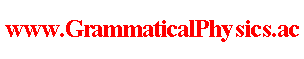since 2006 Help　Sitemap < Forum > < Problems > < What is Renormalization? > < Cartoon > I suppose that renormalization is the third limitation mathematics after differentiation and integration. So, I suppose that the fundamental law of physics is expressed by using renormalization. It is like that already known physical laws are expressed by using differentiation. I have once thought that a theory's needing renormalization means the theory's being wrong. String theorists perhaps have such belief because string theories do not need renormalization. Necessity of renormalization comes from being zero of the size of an elementary particle. It is already seen at classical electrodynamics. String theories do not need renormalization because the size of a string is finite. However, I believe that the core truth tends to hide in annoying unclearly understood things which we do not want to think about it. Renormalizability is often used to judge standability of theories. It also suggests that renormalization is used to write the correct most fundamental theory. I do not deny value of string theories. I suppose that string theories will have meaning as regularization. I think that extra dimensions of spacetime are weak points of string theories for being real. New particle is not justified till it is discovered by experiment. Why are extra dimensions justified by only explaining why they can not be seen? Isn't it that they are not justified till they are seen by experiment? Now I start the main part of the content of this page. As an equation to determine the value of x when the value of y is given, I put the following equation. x(x - a)y = b2 ＞ 0. As an equation to determine the value of y when the value of x is given, I put the following equation. xy = 0. To determine x and y at the same time, we have only to use the following simultaneous equations. x(x - a)y = b2, xy = 0. x is a cartoon of a classical history of a Newtonian particle. y is a cartoon of a set of electromagnetic fields. The first equation is a cartoon of Newtonian equation of motion. The second equation is a cartoon of Maxwell equation. The simultaneous equations are cartoons of classical electrodynamics. a is a cartoon of the mass of the particle. b is a cartoon of the electric charge of the particle. x = 0 is forbidden by x(x - a)y = b2 ≠ 0. If x ≠ 0, y = 0 because xy = 0. However if y = 0, x diverges because x(x - a)y = b2 ≠ 0. This is a cartoon of the problem of infinite reaction received by a particle from electromagnetic fields. Then I change both equations slightly. x[x - f(ε)]y = b2, xy = ε. This is a regularization. Solving these simultaneous equations is as follows. y = ε/x, x[x - f(ε)]ε/x = b2, εx - εf(ε) = b2, x = b2/ε + f(ε), y = ε/[b2/ε + f(ε)]. For example, if f(ε) = a' - b2/ε, (x, y) → (a', 0) as ε → +0. However f(ε) → -∞. This means that bare mass diverges to negative infinity. Negative infinite mass is not physical, so the original equation has no physical meaning. a' is a cartoon of renormalized mass. I suppose that expression of fundamental physical law is defined by using limitation operation other than differentiation and integration. Renormalization is that operation. --- The idea described in this page was posted as a paper to Philosophy of Science Society Japan and rejected. It was in 2002. Author Yuichi Uda, Write start at 2019/10/02/14:28JST, Last edit at 2019/10/04/14:27JST